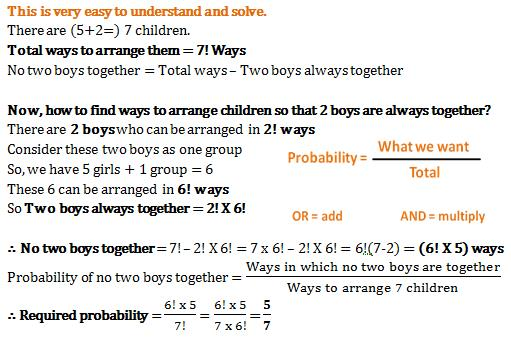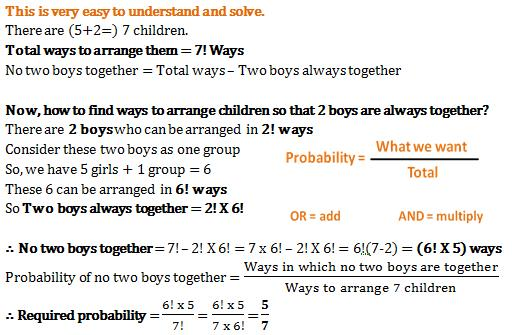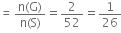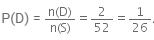# Test: Probability- 3

## 25 Questions MCQ Test Mathematics (Maths) Class 10 | Test: Probability- 3

Description
This mock test of Test: Probability- 3 for Class 10 helps you for every Class 10 entrance exam. This contains 25 Multiple Choice Questions for Class 10 Test: Probability- 3 (mcq) to study with solutions a complete question bank. The solved questions answers in this Test: Probability- 3 quiz give you a good mix of easy questions and tough questions. Class 10 students definitely take this Test: Probability- 3 exercise for a better result in the exam. You can find other Test: Probability- 3 extra questions, long questions & short questions for Class 10 on EduRev as well by searching above.
QUESTION: 1

### If A be the event such that P(A) = 2/5, then P(not A) is equal to

Solution:

We know Probability law which is law of  complement
Which is P(not A)=1-P(A)
Where P(A)=⅖
P(not A)=1-⅖=3/5

QUESTION: 2

### What are the chances that no two boys are sitting together for a photograph if there are 5 girls and 2 boys?

Solution:QUESTION: 3

### In a single throw of a die, the probability of getting a multiple of 3 is

Solution:

Given : A die is thrown once .

A die has 6 faces marked as 1, 2, 3, 4, 5 and 6.

If we throw one die then there possible outcomes are as follows: 1, 2, 3, 4, 5 and 6

Number of possible outcomes are = 6

Let E = Event of getting a getting a multiple of 3

Multiples of 3 are = 3, 6

Number of outcome favourable to E = 2

Probability (E) = Number of favourable outcomes / Total number of outcomes

P(E) = 2/6  = 1/3

Hence, the probability of getting a  multiple of 3, P(E) = 1/3

QUESTION: 4

Find the probability of getting a number greater than 2 when a die is thrown

Solution:

Probability of getting a number greater than 2 is
number greater than 2 = 4
total outcomes = 6
so, 4/6
=2/3

QUESTION: 5

If a die is rolled, the probability of getting a number between 1 and 6 is

Solution:

Between 1 and 6, meaning not 1 or 6, then we have four favorable choices (2, 3, 4, 5) out of six possible outcomes (1, 2, 3, 4, 5, 6). Therefore your probability is 4/6 = 2/3.

QUESTION: 6

What are the chances that no two boys are sitting together for a photograph if there are 5 girls and 2 boys?

Solution:QUESTION: 7

What is the probability of getting one head and one tail when a coin is tossed 2 times?

Solution:

Assume that is an equal chance of the coin landing on heads or tail (the coin is fair, not biased).

Probability of coin landing on head and then on tails=1/2 x 1/2=1/4

Probability of coin landing on tails and then on heads= 1/2 x 1/2 =1/4

Therefore, probability of getting one head and one tail in two coin tosses=1/4+1/4=1/2

QUESTION: 8

What is the probability of getting two heads when a coin is tossed twice?

Solution:
QUESTION: 9

What is the probability of getting no head when two coins are tossed?

Solution:

Two coins are tossed simultaneously, so there are four possible outcomes ie, HH,HT,TT,TH
Total number of outcomes=4
Probability of getting no head=no. of favourable outcomes/ total no. of outcomes
=1/4

QUESTION: 10

In a simultaneous throw of two coins the probability of getting at least one head is

Solution:

Two coins are simultaneously tossed.
So sample space={HH,HT,TH,TT}
No. of favourable outcomes=getting at least one head={HH,HT,TH}
=3
Total number of outcomes=4
Probability of getting at least one head=No. of favourable outcomes/Total number of outcomes
=3/4

QUESTION: 11

The probability of getting a jack card is

Solution:

Total number of outcomes = 52
Favourable outcomes = four cards are of Jack.
Total number of favourable outcomes = 4

Probability = Number of favourable outcomes/ Total number of outcomes.

Required probability = P(jack) = 4/52= 1/13.

Hence, the probability of getting Jack is 1/13.

QUESTION: 12

The probability of getting a face card is

Solution:

Total Cards: 52

Total Face cards: 12 (4 Jacks, 4 Queens, 4 Kings)

P(Choosing a Face card) =12/52= 3/13

QUESTION: 13

One card is drawn from a pack of 52 cards, each of the 52 cards being equally likely to be drawn. Find the probability that the card drawn is :

The probability of getting a '10' of black suit is

Solution:

Let G be the favourable outcomes of getting 10 of black suit then

⇒ n(G) = 2

Therefore,  P(G)QUESTION: 14

One card is drawn from a pack of 52 cards, each of the 52 cards being equally likely to be drawn. Find the probability that the card drawn is :

The probability of getting a red and a king card is

Solution:

Let D be the favourable outcomes of getting red and a king, then n(D)=2 Therefore,QUESTION: 15

A bag contains 4 red balls and 3 green balls. A ball is drawn at random. The probability of drawing a green ball is

Solution:

Total number of outcomes=7
No. of favourable outcomes=3 (no. Of green balls)
Probability of getting a green ball=No. Of favourable outcomes/Total no. of outcomes=3/7

QUESTION: 16

P(E) + P(E)¯ is equal to

Solution:
QUESTION: 17

Which one of the following cannot be the probability of an event

Solution:
QUESTION: 18

Which one of the following cannot be the probability of an event

Solution:

Probability of an event can only be a proper fraction and not an improper fraction.i.e.the numerator cannot be greater than the denominator.
P(E)=Number of outcomes favourable to E/Number of all possible outcomes of the experiment.

QUESTION: 19

Probability of an impossible event is equal to

Solution:
QUESTION: 20

If P(E1) = 1/6, P (E2) = 1/3 , P (E3) = 1/6 , where E1, E2, E3 and E4 are elementary events of a random experiment, then P (E4) is equal to

Solution:
QUESTION: 21

Cards each marked with one of the numbers 4, 5, 6,...., 20 are placed in a box and mixed thoroughly. One card is drawn at random from the box. Then, the probability of getting an even prime number is

Solution:

We have one and only even prime number which is 2. So no. of favourable outcomes= 0  and total no. of outcomes=17
Probability of getting 2 =0 this means that 2 cannot occur .

QUESTION: 22

A bag contains 5 red and 4 black balls. A ball is drawn at random from the bag. Then, the probability of getting a black ball is

Solution:
QUESTION: 23

Find the probability of getting a head in a throw of a coin.

Solution:
QUESTION: 24

Two fair coins are tossed simultaneously. Find the probability of Getting only one head

Solution:

When two coins are tossed, the sample space of possible outcomes would be the set

S = {HH, HT, TH, TT}

where H: the outcome is a “Head” and T: the outcome is a “Tail” and the probabilities associated with each of these outcomes are equal assuming the coins are fair.

When we say at most 1 “Head” outcome, it means that only 1 of the two coins show up a head or that the no “Head” outcome shows up.

If A is the event obtaining only 1 “Head” outcome, then the set of outcomes that makes up event A is

A = {(HT), (TH)}

Hence, the probability of event A is

P(A) = 2/4

QUESTION: 25

Two fair coins are tossed simultaneously. Find the probability of Getting atleast one head

Solution:

When two coins are tossed simultaneously then the possible outcomes obtained are {HH, HT, TH, and TT}.

Here H denotes head and T denotes tail.

Therefore, a total of 4 outcomes obtained on tossing two coins simultaneously.

Consider the event of obtaining at the most one head. At most one head is obtained means either no head is obtained or one head is obtained.

The outcome(s) favourable to no head obtained is {TT}

The outcome(s) favourable to one head obtained is {HT, TH}

Therefore, the event of obtaining at most one head has 3 favourable outcomes. These are TT, HT and TH.

Therefore, the probability of obtaining at most one head = 3/4.